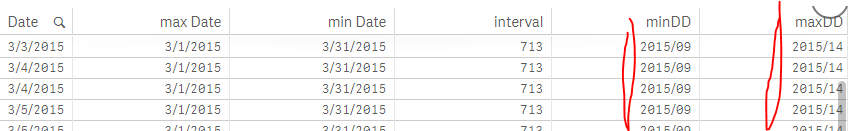# App Development

Announcements
Skip the ticket, Chat with Qlik Support instead for instant assistance.
cancel
Showing results for
Did you mean:Not applicable

## Condition based on Drill Down level in Qlik Sense

Hello,

I would need some help with following logic:

I have a drill down date dimension (year/month/week/day) and I want to compare values (for example sum of sold products) at the begining and end of some period. The issue is, that I want to compare sum(sold_products) in min month and max month, THEN, when I choose only one month, I drill down to weeks and here aI want to compare sum(sold_products) in min week and max week.

Lowest level in Date dimension is Time, so I've tried to use Interval() to find out, where in Drill Down dimension I'm right now:

minDD:

if(Interval(Max(total Date)-Min(total Date), 'hh') < 24, DayName(Min(total Date)),

if(Interval(Max(total Date)-Min(total Date), 'hh') > 24, if(Interval(Max(total Date)-Min(total Date), 'hh')

< 168, WeekName(Min(total Date)), MonthName(Min(total Date)))))

maxDD:

if(Interval(Max(total Date)-Min(total Date), 'hh') < 24, DayName(Max(total Date)),

if(Interval(Max(total Date)-Min(total Date), 'hh') > 24, if(Interval(Max(total Date)-Min(total Date), 'hh') < 50, WeekName(Max(total Date)),

MonthName(Max(total Date)))))When I choose one month, so I drill down to weeks, minDD and maxDD show weeks:Now I want to use this logic in KPIs.

I want to compare last and first Month/Week/Day.

For example this works for Days:

SUM(

if(Interval(Max(total Date)-Min(total Date), 'hh') < 24,

IF(DayName(Date) = DayName(Min(total Date))

,Sales)))

But I cannot figure out, how to implement the rest. For example when I tried to add Weeks, expression dosn't work:

SUM(
if(Interval(Max(total Date)-Min(total Date), 'hh') < 24,
IF(DayName(Date) = DayName(Min(total Date)),

if(Interval(Max(total Date)-Min(total Date), 'hh') > 24,
if(Interval(Max(total Date)-Min(total Date), 'hh') < 168,
IF(WeekName(Date) = WeekName(Min(total Date))

,Sales))))))

Thanks,

Mirek

1 Solution

Accepted SolutionsNot applicable
Author

Soo....

Seams like I found solution using Pick() and Match() function:

Pick(

Match(

IF(Interval(Max(total Date)-Min(total Date), 'D')<8,'Days',

IF(Interval(Max(total Date)-Min(total Date), 'D')>7,

IF(Interval(Max(total Date)-Min(total Date), 'D')<30,'Weeks','Months')))

,'Days','Weeks','Months'

),

sum(IF(DayName(Date) = DayName(Max(total Date)),Sales)),

sum(IF(WeekName(Date) = WeekName(Max(total Date)),Sales)),

sum(IF(MonthName(Date) = MonthName(Max(total Date)),Sales))

)

I'm still not sure about number of days in Interval() condition, but I will focus on this later.

I hope it helps someone 😉

MiroslavNot applicable
Author

Soo....

Seams like I found solution using Pick() and Match() function:

Pick(

Match(

IF(Interval(Max(total Date)-Min(total Date), 'D')<8,'Days',

IF(Interval(Max(total Date)-Min(total Date), 'D')>7,

IF(Interval(Max(total Date)-Min(total Date), 'D')<30,'Weeks','Months')))

,'Days','Weeks','Months'

),

sum(IF(DayName(Date) = DayName(Max(total Date)),Sales)),

sum(IF(WeekName(Date) = WeekName(Max(total Date)),Sales)),

sum(IF(MonthName(Date) = MonthName(Max(total Date)),Sales))

)

I'm still not sure about number of days in Interval() condition, but I will focus on this later.

I hope it helps someone 😉

MiroslavTags
Community Browser